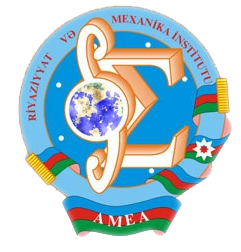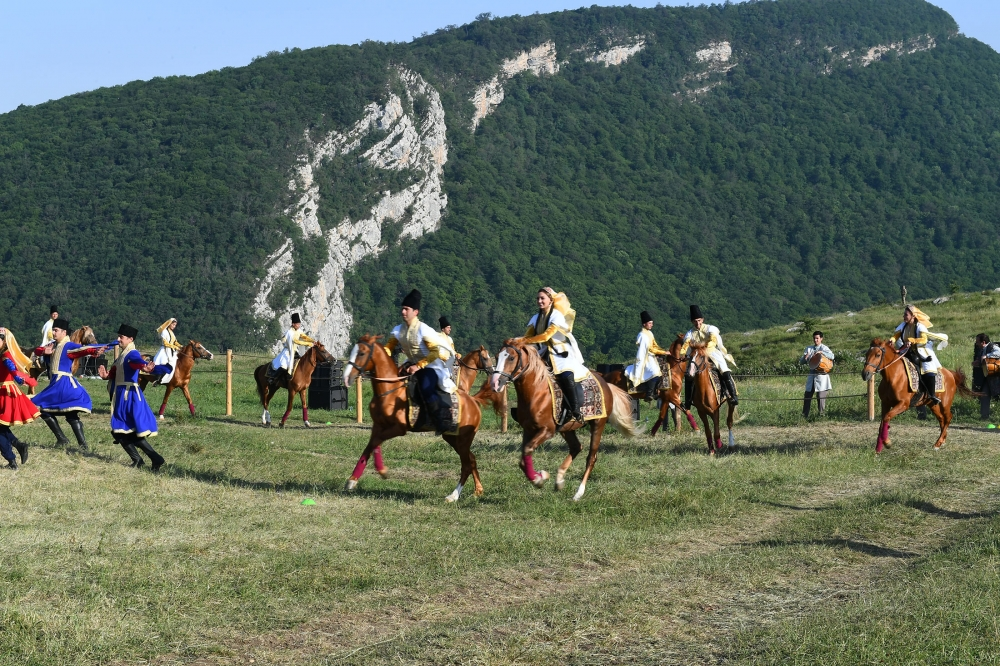Riyaziyyat və Mexanika İnstitutu

## Qarabağ xəbərləri### Şuşada Heydər Əliyev Fondunun təşkilatçılığı ilə “Musiqi irsi və Qarabağ atları Cıdır düzündə” adlı kompozisiya təqdim olunub## Proceedings 2012/37/XLV

 Year: 2012 Volume: XXXVII Number: XLV MATHEMATICS Aliev B.A. Abstract PDF A boundary value problem for second order elliptic differential-operator equations with a spectral parameter and discontinuous coefficient [PDF] 3 Aliyev O.S., Bayramova N.Q. Abstract [PDF] Behavior of solutions of degenerated parabolic equations [PDF] 21 Akhundova E.B. Abstract [PDF] Representation for eigen functions of third order differential operator [PDF] 25 Azizov F.J., Abdurakhmanov V.A.,Khalilov V.S. Abstract [PDF] Asymptotics of numerical characteristics of the first passage time of level by the trajectory of perturbed Markov chain [PDF] 33 Eroglu A., Alizadeh F.Ch. Abstract [PDF] Boundedness of fractional integral operators with rough kernel on generalized Morrey spaces [PDF] 39 Guliyev V.S. Rahimova K.R. Abstract [PDF] Parabolic fractional maximal operator in parabolic generalized Morrey spaces [PDF] 51 Hashemi M., Hashemi R. Abstract [PDF] On the commuting graphs of finitely generated groups [PDF] 67 Khalilov E.H. Abstract [PDF] Cubic formula for the potential of a simple layer and its normal derivative [PDF] 77 Mamedova F.M. Abstract [PDF] A Hardy type variable exponent inequality for the decreasing exponents [PDF] 85 Mamedov E.M. Abstract [PDF] On the stabilization of solutions for pseudohyperbolic equation with nonlinear boundary conditions [PDF] 89 Mamedov F.I., Amanov R.A. Abstract [PDF] On the removable singularity of solutions of nonlinear elliptic equations [PDF] 95 Musayev H.K. Abstract [PDF] A priori estimations for coercive solvability of differential-operator equations with variable coefficients [PDF] 111 Yusufov V.Sh. Abstract [PDF] Cardinal invariants and semigroups of homeomorphic mappings of topological spaces [PDF] 117 APPLIED PROBLEMS OF MATHEMATICS AND MECHANICS Guliyev H.F., Nazarova V.B. Abstract [PDF] A problem an elastic bar vibrations control [PDF] 121 Seyfullayeva H.I. Abstract [PDF] Thickness optimization of an oscillating plate-like construction [PDF] 129 Tagiyev H.T. Abstract [PDF] Gradient of the functional in the optimal control problem with non-local conditions for the wave equation [PDF] 139
Azərbaycanda COVID-19 ilə bağlı statistika
• Virusa yoluxan

596973

• Sağalan

565521

• Yeni yoluxan

0

• Aktiv xəstə

23469

• Ölüm halı

7983

• Test edilib

5,608,158